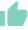# PB RATIO - Meaning, Formula, Calculation, ExampleGaurav Seth
20 Likes | 763 days agoLikeShareAuthor is a Zfunds Verified ExpertGaurav Seth
Gurugram## WHAT IS PB RATIO?

The PB Ratio i.e. Price to Book ratio measures a company’s market price with respect to its book value. This book value refers to a company’s financial worth derived by calculating the difference between all its assets and expenses along with debts whereas the market value refers to the entity’s market capitalization. It is based on the current market price of shares multiplied by the number of shares outstanding.

## SIGNIFICANCE OF PB RATIO

The PB ratio indicates whether an entity’s asset value is comparable to the market price of its share or not. It can be used very effectively for finding the value stock especially when companies that are composed of mostly liquid assets such as investment, insurance, finance, and banking firms.  Though this ratio is not useful for firms with large Research & Development expenditures or firms with high levels of property or other assets.

Just like any other ratio, it is best to compare the PB ratio within specific industries. For instance,  financial stocks mostly trade below book value whereas technology shares often trade above book value.

## FORMULA AND CALCULATION OF PB RATIO

The formula for the price-to-book ratio is stated below:

PB Ratio = Share price/[book value per share or (asset - liabilities)/outstanding share}]

The calculation process is as stated below:

1. Get the current market price of the share. You can get it simply by searching on google or your investing application.
2. Ascertain the BVPS (Book Value Per Share). You can find it by scrolling and looking at the financial stock listing site.
3. Now you have to divide the share price by the BVPS. And you will get your PB ratio.

## WORKING AND EXAMPLE OF PB RATIO

Let’s take a company named ABC limited whose share is trading at Rs. 6 per share and there are 100 outstanding shares.
Here is a balance sheet abstract for company ABC

Here, the book value will be

= 2,000 - 1,500 = 500

Book value per share: 500/100 = Rs.5

PB ratio = Stock Price/BVPS

= 6/5
= 1.2

PB Ratio of less than 1 signifies that a stock is currently undervalued, while a ratio more than 1 may indicate that a stock is overvalued.

PB Ratio, identical to PE ratio, gives an easily understandable position of the company’s valuation with respect to its net worth. It is more stable as asset prices are not as volatile as earnings and can also be used to value entities that have not earned revenues that are startups and liquidating companies.

1. Because this ratio is based on accounting recognition of assets, it does not capture the true and fair value of all the assets because the accounting principles have not yet evolved to value intangible assets. While book values might have been significantly relevant in the past, the relevance is declining in the modern era when the proportion of intangible assets is booming. The prime example of this are e-commerce or digital-based entities. So, the companies having a greater portion of intangible assets will see an optically higher PB ratio than those having equal amounts of tangible assets.
2. Another limitation of this ratio is that the value of assets is subject to the discretion of administration and management because entries like goodwill and depreciation can be easily manipulated to either increase or decrease the asset book value.
3. This ratio cannot be implemented on entities having a net worth in negative. For instance, Spicejet is currently having a net worth in negative and this can be the case with many startups and mature companies as well which are going through a tough patch.

FAQs

1. What is PB Ratio?

The PB Ratio i.e. Price to Book ratio measures a company’s market price with respect to its book value per share.

2. Why does PB Ratio matter?

This ratio matters because it can be used very effectively for finding value stock especially when companies that are composed of mostly liquid assets such as investment, insurance, finance, and banking firms.

3. What is the formula to calculate PB Ratio?

The formula to calculate PB ratio is:

PB Ratio = Share price/[book value per share i.e (asset - liabilities)/outstanding share}]

4. What is the key advantage of the PB Ratio?

The key advantage of the PB Ratio is that it gives an easily understandable position of the company’s valuation with respect to its net worth and it is more stable.

5. What are the limitations of the PB Ratio?

The limitation of this ratio is that it may not be true and fair and can not be implemented on entities having negative net worth.